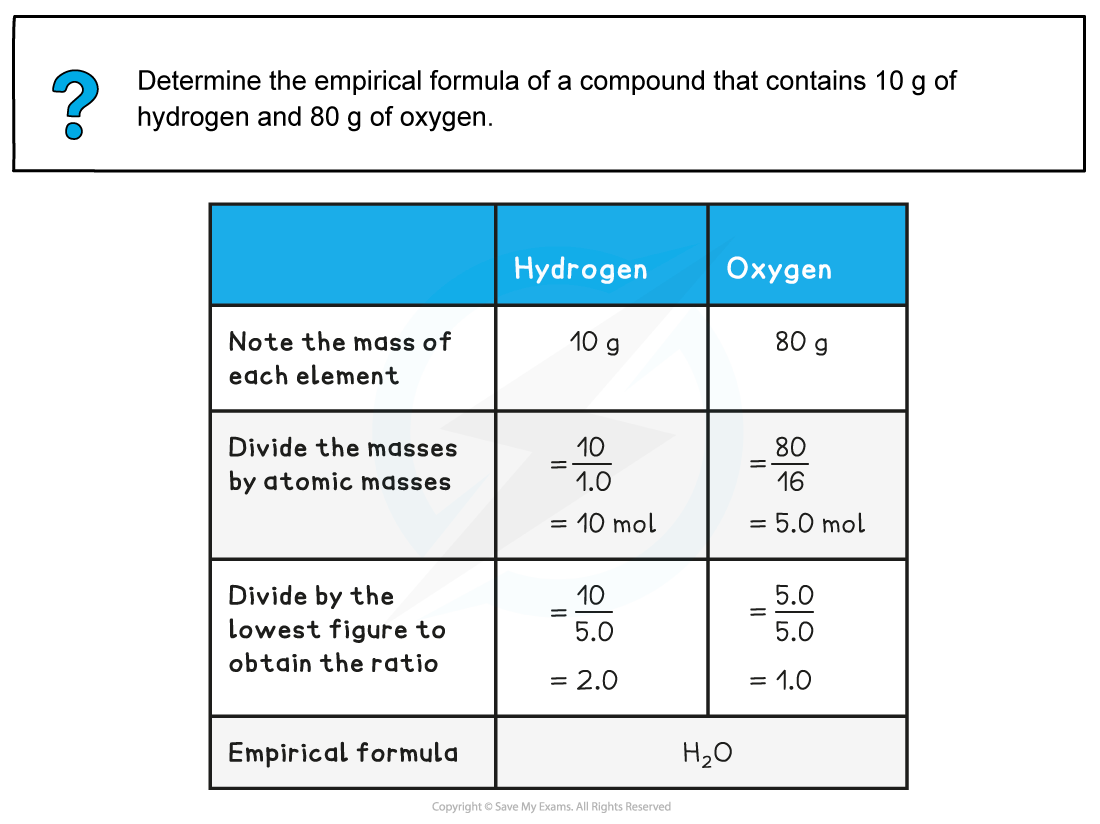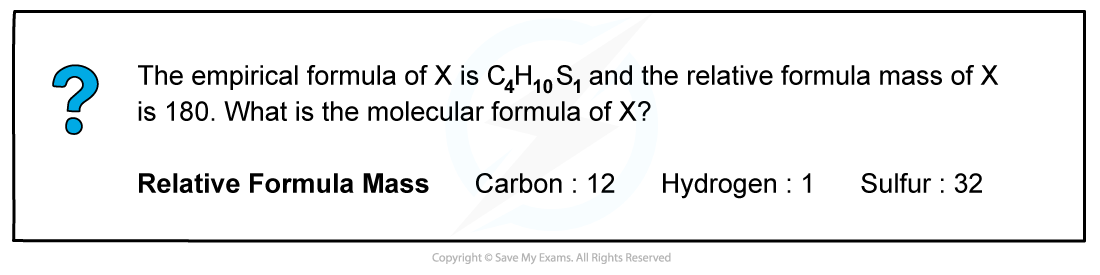# CIE A Level Chemistry复习笔记1.2.5 Empirical & Molecular Formulae

### Empirical & Molecular Formulae

• The molecular formula is the formula that shows the number and type of each atom in a molecule
• Eg. the molecular formula of ethanoic acid is C2H4O2
• The empirical formula is the simplest whole number ratio of the elements present in one molecule or formula unit of the compound
• Eg. the empirical formula of ethanoic acid is CH2O
• Organic molecules often have different empirical and molecular formulae
• Simple inorganic molecules however have often similar empirical and molecular formulae
• Ionic compounds always have similar empirical and molecular formulae

### Empirical & Molecular Formulae Calculations

#### Empirical formula

• Empirical formula is the simplest whole number ratio of the elements present in one molecule or formula unit of the compound
• It is calculated from knowledge of the ratio of masses of each element in the compound
• The empirical formula can be found by determining the mass of each element present in a sample of the compound
• It can also be deduced from data that give the percentage compositions by mass of the elements in a compound

#### Worked Example: Empirical formula from mass#### Worked Example 2: Empirical formula from %#### Molecular formula

• The molecular formula gives the exact numbers of atoms of each element present in the formula of the compound
• The molecular formula can be found by dividing the relative formula mass of the molecular formula by the relative formula mass of the empirical formula
• Multiply the number of each element present in the empirical formula by this number to find the molecular formula

#### Worked example: Calculating molecular formula• Step 1: Calculate relative formula mass of empirical formula

Relative formula mass = (C x 4) + (H x 10) + (S x 1)

Relative formula mass = (12 x 4) + (1 x 10) + (32 x 1)

Relative formula mass = 90

• Step 2: Divide relative formula mass of X by relative formula mass of empirical formula

Ratio between Mr of X and the Mr of the empirical formula = 180/90

Ratio between Mr of X and the Mr of the empirical formula = 2

• Step 3: Multiply each number of elements by 2

(C4 x 2) + (H10 x 2) + (S1 x 2)     =    (C8) + (H20) + (S2)

Molecular Formula of X is C8H20S2